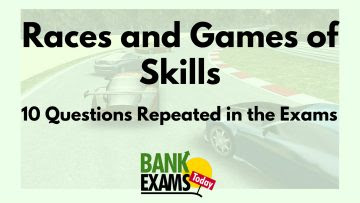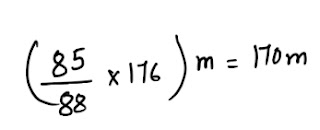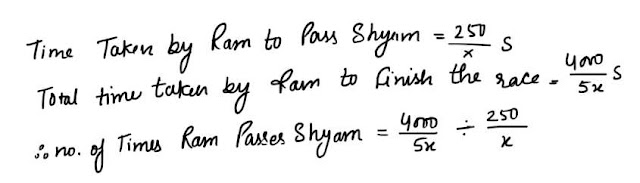# Races and Games : 10 Questions that are Repeated in the Exams

Races and Games of skills is an important chapter for banking examinations point of view. Here on BankExamsToday.com I am analyzing various exam question papers from past many years. Today I am listing down 10 questions that repeated in almost all the exams.#### Ques 1 .

In a kilome.tre race, A beats B by 30 metres or 6 seconds . Find the time taken by A to finish the race.
Ans 1. It is abvious that B runs 30 metres in 6 seconds .Time taken by A to finish the race = 200-=6 = 194 seconds = 3 minutes 14 seconds

#### Ques 2.

In a 125 m Race , A runs at 10 km/hour. A gives B a start of 5 metres and still beats him by 5 seconds . Find the speed of B.
Ans 2.#### Ques 3.

In a kilometre race, if A gives B 30 m start, A wins by 20 seconds but if A gives B 30 seconds start, B wins by 20 m. Find the speed of B.
Ans 3. Let the speed of A be x m/s and speed of B y m/s.Hence speed of B = 4.75 m/s.

#### Ques 4.

A can run a kilometre race in 2 minutes and B in 2 minutes. by what distance can A beat B ?
Ans 4. A beats B by 40 sec.A beats B by 20 metres .

#### Ques 5.

In a 100 m race , A beats B by 12 m and C by 15 m , In a race of 176 m, Find by how much distance would B beat C.
Ans 5. When A covers 100 m, B covers 88 m and C covers 85 m.
i.e. when B covers 88 m C covers 85 m.
when B covers 176 m, C would coverB would beat C by 6 m in a race of 176 m.

#### Ques 6.

In a race of 600 m, A can beat B by 50 m and in a race of 500 m, B can beat C by 40 m . by how many metres will A beat C in a race of 400 m?
Ans 6. When A covers 600 m , B covers 550 m.#### Ques 7.

In a 400 m. race , the ratio of speeds of two runners A and B is 3:4. a Has a start of 130 m. A wins by
(a) 50 m
(b) 25 m
(c) 40 m
(d) 75 m
Ans 7. 40 m.#### Ques 8.

In a 100 m race , P runs at 8 km/hour . If P gives Q a start of 4 m and still beats him by 15 seconds , the speed of Q is
(a) 6 km/hour
(b) 5.5 km/hour
(c) 6.2 km/hour
(d) 5.76 km/hour
Ans 8. 5.76 km/hour#### Ques 9.

Ram and shyam  run at 4 km on a course 250 m round . If their rates be 5 : 4 , how often does the winner pass the other?
(a) Ram passes Shyam thrice
(b) Ram passes Shyam twice
(c) Ram passes Shyam once
(d) Shyam passes Ram twice
Ans 9. Ram passes Shyam thrice
Hint : Speed  of Ram with respect to shyam= 5x - 4x = x ms -1#### Ques 10.

P can run one kilometre in half  a minute  less time than Q. In a kilometre race , Q gets a start of 100 m  and still losses by 100 m. Find  the time P and Q take to run a kilometre .
(a) 3 min , 2 min
(b) 31/2  min , 2 min
(c)2 min , 2 1/2 min
(d) 2 1/2 ,min, 4 min
Ans 10.  2 min , 2 1/2 min
Hint : Let the speed of P and Q be P m/s and q  m/s respectively .#### Free Notes